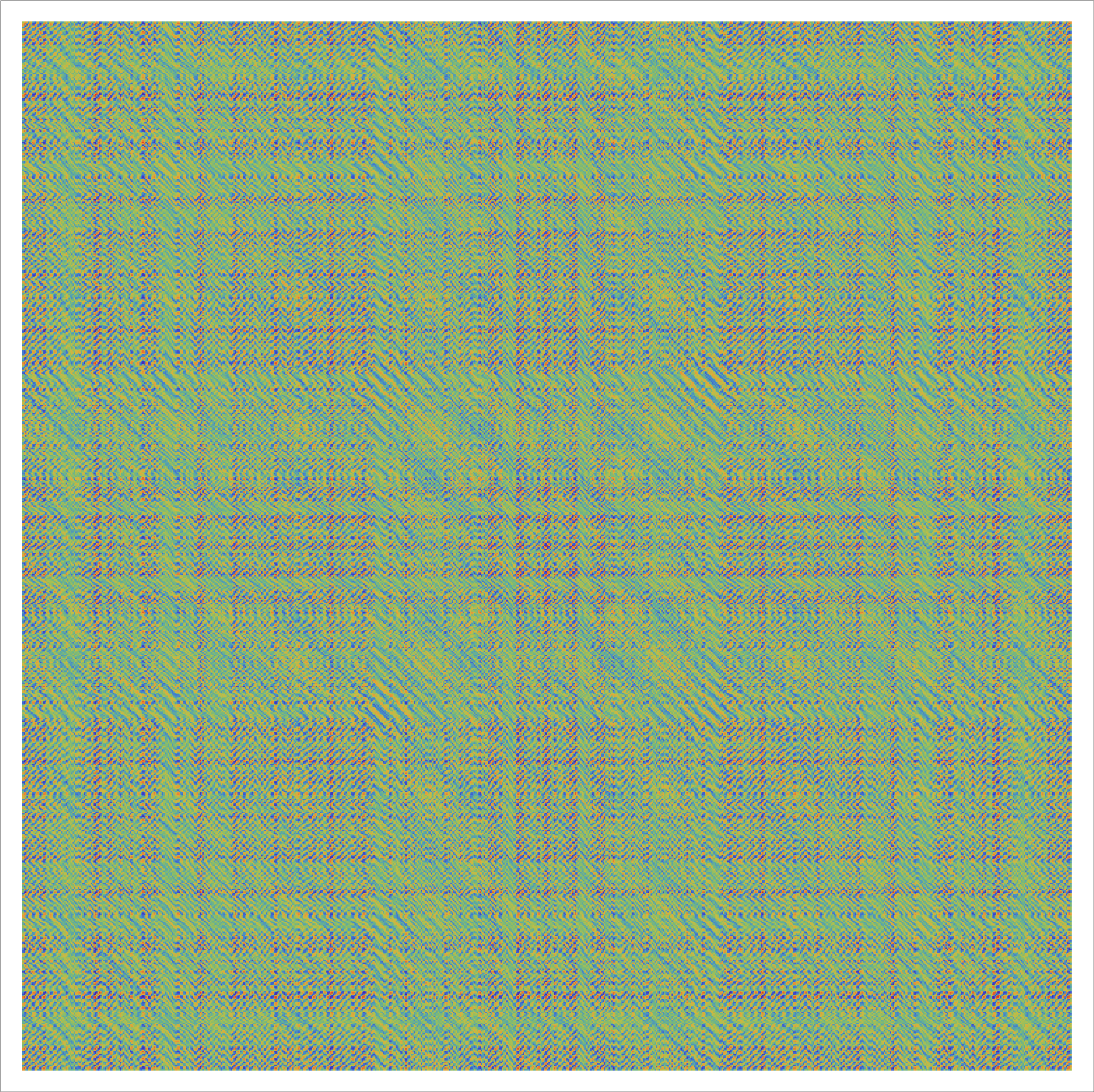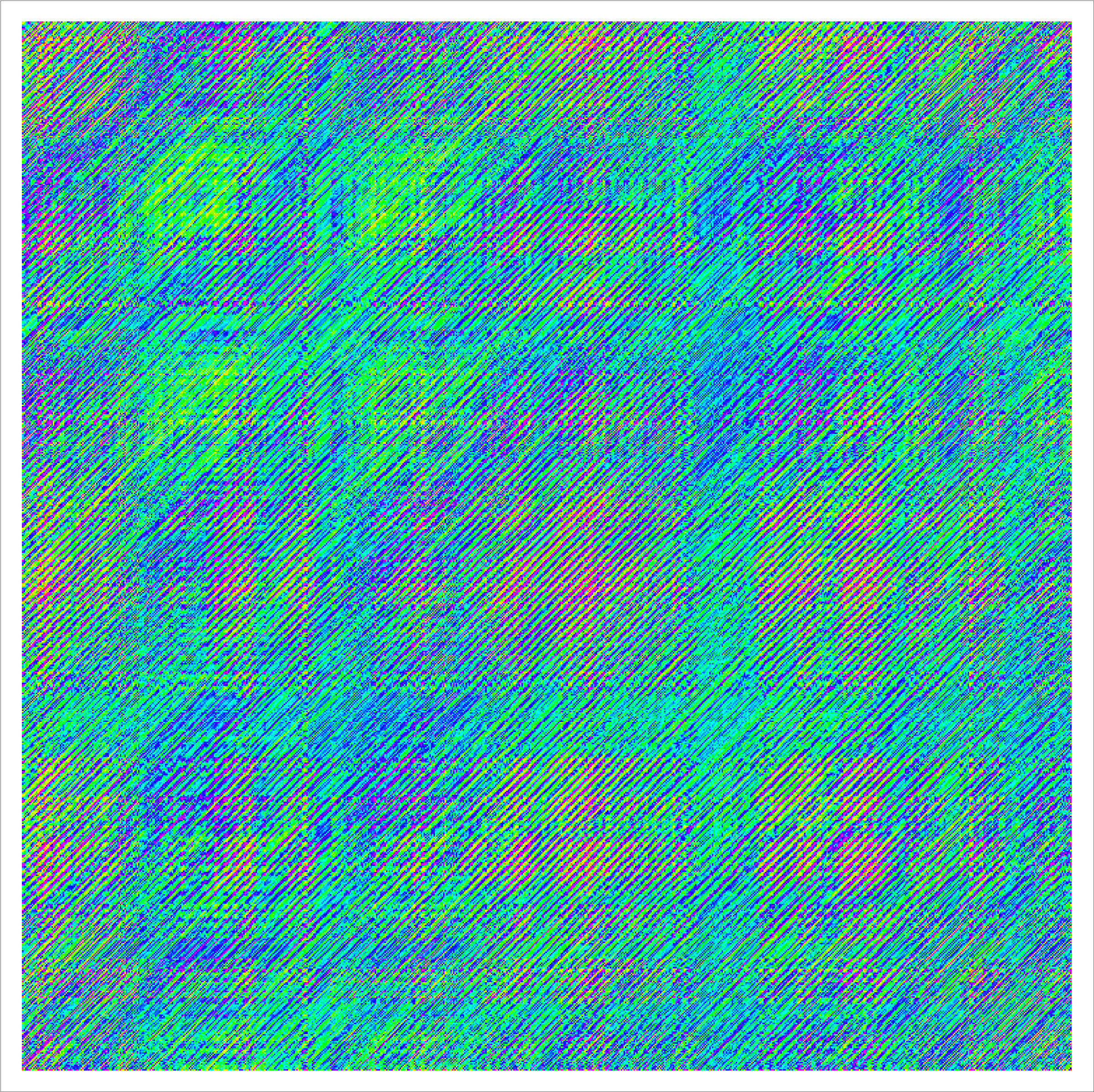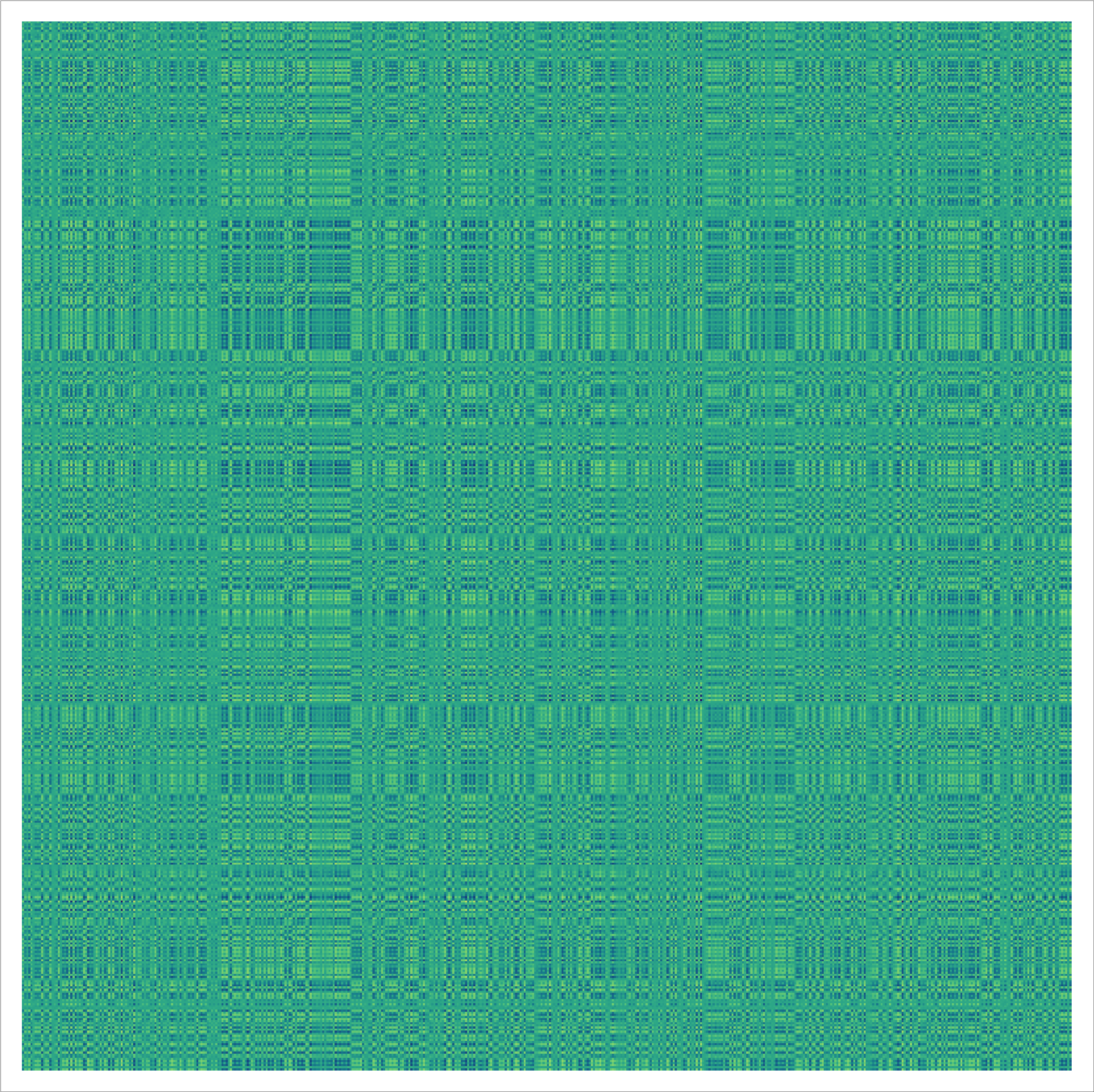# Prime Matrix Art, Jackson Pollock style

Here is the inverse of the 1500 * 1500 matrix which is A(i,j) = 1 if i-j is prime and 0 else.Here is the inverse of the 1500 * 1500 matrix which is A(i,j) = 1 if i+j is prime and 0 else.Here is the inverse of the 500 * 500 matrix which is A(i,j) = 1 if the complex number i + Sqrt(-1) j is prime and 0 else.Is something known about this? No! It is very unlikely that anybody ever even has looked at these inverse matrices before! But it passes as Jackson Pollock art!